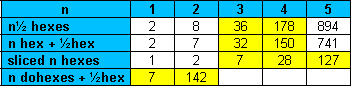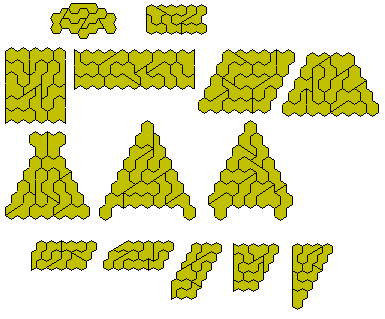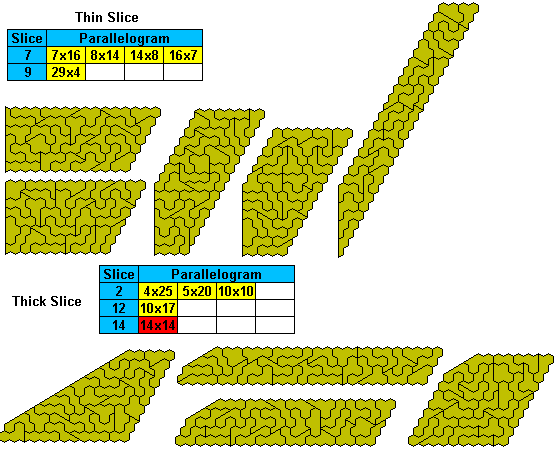Sliced Polyhexestwo-sidedone-sidedVarious possible constructions can be made with sliced polyhexes. Apart from a 'parallelogram' of area ab trapezia and two type of sliced parallelogram are possible. The areas of these figures are shown below. (In the tables axb where a<b refers to the thin slice type A and axb with a>b refers to type B)There are seven sliced trihexes and 12 in the one-sided set. The bottom five figures are the only sliced parallelograms possible.The 28 sliced tetrahexes can make a number of figures.A number of sliced 'parallelograms' are also possible.The one-sided set consists of 54 pieces.A number of sliced 'parallelograms' are also possible.Adding a half hexagon to a dohex gives a set of seven pieces or thirteen pieces with the one-sided set.Adding four hexagons to a half hexagon gives us a set of 178 pieces

.Adding a half hex to the didohexes gives us a set of 142 pieces one of which has a hole. The remainder are shown here.If we add two half hexagons to a single hexagon we get a set of 22 pieces for the two-sided and 35 for the one-sided set. Both sets have an odd number of pieces where the sliced edges are inclined to one another restricting the type of construction which can be made. For example the two-sided set can form the trapezium at the bottom right but cannot form a parallelogram.